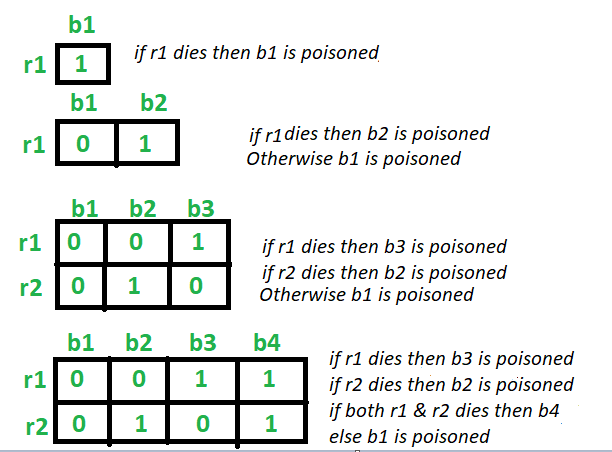Rat and Poisoned bottle Problem

• Difficulty Level : Medium
• Last Updated : 06 Jun, 2021

Given N number of bottles in which one bottle is poisoned. So the task is to find out minimum number of rats required to identify the poisoned bottle. A rat can drink any number of bottles at a time.

Examples:

Input: N = 4
Output: 2

Input: N = 100
Output: 7

Approach:
Let’s start from the base case.

• For 2 bottles: Taking one rat (R1). If the rat R1 drinks the bottle 1 and dies, then bottle 1 is poisonous. Else the bottle 2 is poisonous. Hence 1 rat is enough to identify
• For 3 bottles: Taking two rats (R1) and (R2). If the rat R1 drinks the bottle 1 and bottle 3 and dies, then bottle 1 or bottle 3 is poisonous. So the rat R2 drinks the bottle 1 then. If it dies, then the bottle 1 is poisonous, Else the bottle 3 is poisonous.
Now if the rat R1 does not die after drinking from bottle 1 and bottle 3, then bottle 2 is poisonous.
Hence 2 rats are enough to identify.

• For 4 bottles: Taking two rats (R1) and (R2). If the rat R1 drinks the bottle 1 and bottle 3 and dies, then bottle 1 or bottle 3 is poisonous. So the rat R2 drinks the bottle 1 then. If it dies, then the bottle 1 is poisonous, Else the bottle 3 is poisonous.
Now if the rat R1 does not die after drinking from bottle 1 and bottle 3, then bottle 2 or bottle 4 is poisonous. So the rat R1 drinks the bottle 2 then. If it dies, then the bottle 2 is poisonous, Else the bottle 4 is poisonous.
Hence 2 rats are enough to identify.

• For N bottles:

Minimum number of rats required are = ceil(log2 N))Below is the implementation of the above approach:

C++

 // C++ program to implement // the above approach   #include using namespace std;   // Function to find the minimum number of rats int minRats(int n) {     return ceil(log2(n)); }   // Driver Code int main() {     // Number of bottles     int n = 1025;       cout << "Minimum " << minRats(n)          << " rat(s) are required"          << endl;       return 0; }

Java

 // Java program to implement // the above approach class GFG {     public static double log2(int x)     {         return (Math.log(x) / Math.log(2));     }       // Function to find the minimum number of rats     static int minRats(int n)     {         return (int)(Math.floor(log2(n)) + 1);     }           // Driver Code     public static void main (String[] args)     {         // Number of bottles         int n = 1025;               System.out.println("Minimum " + minRats(n) +                            " rat(s) are required");     }    }   // This code is contributed by AnkitRai01

Python3

 # Python3 program to implement # the above approach import math   # Function to find the # minimum number of rats def minRats(n):           return math.ceil(math.log2(n));   # Driver Code   # Number of bottles n = 1025; print("Minimum ", end = "") print(minRats(n), end = " ") print("rat(s) are required")   # This code is contributed # by divyamohan123

C#

 // C# program to implement // the above approach using System;   class GFG {     public static double log2(int x)     {         return (Math.Log(x) / Math.Log(2));     }       // Function to find the minimum number of rats     static int minRats(int n)     {         return (int)(Math.Floor(log2(n)) + 1);     }           // Driver Code     public static void Main (String[] args)     {         // Number of bottles         int n = 1025;               Console.WriteLine("Minimum " + minRats(n) +                           " rat(s) are required");     } }   // This code is contributed by 29AjayKumar

Javascript



Output:

Minimum 11 rat(s) are required

My Personal Notes arrow_drop_up
Recommended Articles
Page :